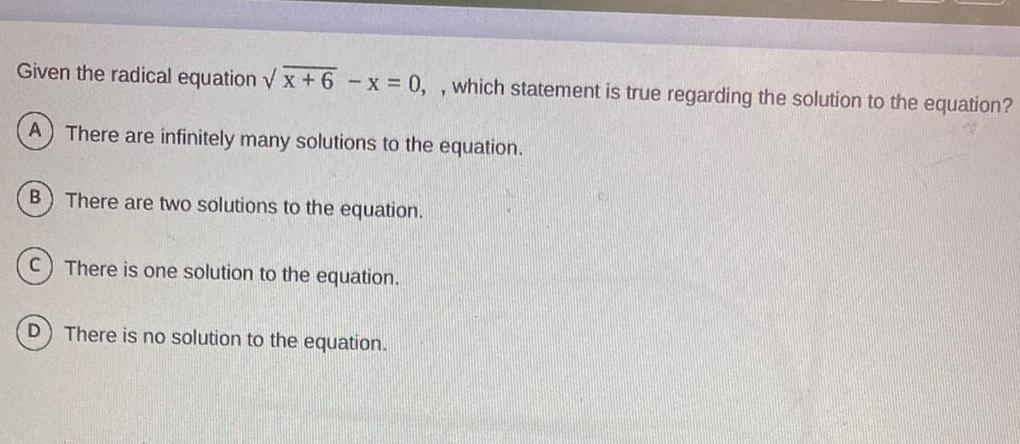Question:

# Given the radical equation x 6 x 0 which statement is true

Last updated: 3/9/2023Given the radical equation x 6 x 0 which statement is true regarding the solution to the equation A There are infinitely many solutions to the equation B D There are two solutions to the equation There is one solution to the equation There is no solution to the equation T